# Civil Engineering - Surveying - Discussion

### Discussion :: Surveying - Section 1 (Q.No.16)

16.

If a 30 m chain diverges through a perpendicular distance d from its correct alignment, the error in length, is

 [A].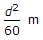[B].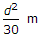[C].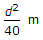[D].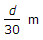[E].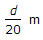.

Explanation:

No answer description available for this question.

 Anand Singh said: (May 8, 2014) Slope correction= h^2/2D = d^2/2*30 = d^2/60.

 Nagaraju said: (Jul 29, 2014) How can we determine this can you explain in detail?

 Swapnil said: (Sep 23, 2014) Is 'h' equals to 'd'?

 Saubhagya Laxmi said: (Jan 24, 2015) Unable 2 get your explanation.

 Mahesh Parajuli said: (Feb 24, 2015) h^2/(2L). where L = Chain length. h = Max deflection.

 Atul said: (Feb 25, 2015) As slope correction formula is h2/2d its in general chain surveying correction.

 Ashutosh said: (Oct 24, 2015) Alignment correction formula is (h^2)/2L , where h is error from correct alignment.

 Preety said: (Apr 23, 2016) h^2/2L is the formula of alignment correction. = h^2/2*30. = h^2/60.

 Aadil said: (Jun 21, 2017) For bad ranging or misalignment. C= d^2/2l.

 Ramya said: (Jun 29, 2017) I didn't get this, please explain me.

 Ravi Kumar said: (Jul 6, 2017) I can't understand please explain me.

 Er Meghanada (Bhadrak ) said: (Nov 12, 2017) Formula Of Alignment correction = h Square /2L, Length of Chain (L)= 30 m. = h Square / 2*30 m = h Square / 60 m. (Right Answer = d square / 60 m).

 Sammy said: (Apr 29, 2018) For example, You are on the sloped surface and you are measuring horizontal distances, so here one end is fixed and when you move to the other end on a sloped surface, there will be the difference in elevations from two ends of the chain, NOW, HERE WILL COME ERROR BECAUSE OF THE EARTHS CURVATURE, and that error is cumulative (an error will add with slope length). so the formula is h2/2l = d2/2*30 = d2/60 , (d= verticle distance)

 Anurag Jain said: (Feb 6, 2019) h^2/2L is the formula of alignment correction. = h^2/2*30. = h^2/60.

 Pavan said: (Mar 15, 2019) Thank you. @Anand Singh.

 Goutham said: (May 5, 2019) Misalignment is d^2/L= d^2/30 is the right answer.

 Pranay said: (May 20, 2019) Correction for misalignment is d^2/l.

 Saad said: (Jul 1, 2019) H √2*l.

 Manzoor Hussain said: (Jun 30, 2020) Thank you @Sammy.

 Pankaj Prajapati said: (Sep 20, 2020) Alignment correction = h^2/2L = d^2/2 * 30 = d^2/60.

 Satya said: (Nov 4, 2020) It is H^2/60.